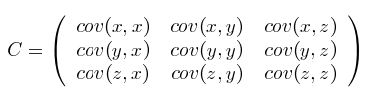• 均值：$$\bar{X}=\frac{\sum_{i=1}^n X_{i}}{n}$$
• 标准差：$$s=\sqrt{\frac{\sum_{i=1}^n (X_{i}-\bar{X})^2}{n-1}}$$
• 方差：$$s^2=\frac{\sum_{i=1}^n (X_{i}-\bar{X})^2}{n-1}$$

$var(X)=\frac{\sum_{i=1}^n (X_{i}-\bar{X})(X_{i}-\bar{X})}{n-1}$

$cov(X,Y)=cov(Y,X)=var(X)=\frac{\sum_{i=1}^n (X_{i}-\bar{X})(Y_{i}-\bar{Y})}{n-1}$

$C_{n\times n}=(c_{i,j},c_{i,j}=cov(Dim_{i},Dim_{j}))$# I. MATLAB 实现微信支付宝